# WHAT IS CONJUGATE AND RECTANGULAR OR EQUILATERAL HYPERBOLA- CONCEPT WITH EXAMPLE

CONJUGATE AND RECTANGULAR OR EQUILATERAL HYPERBOLA

CONJUGATE HYPERBOLA :

Two hyperbolas such that transverse & conjugate axes of one hyperbola are respectively the conjugate & the
transverse axes of the other are called Conjugate Hyperbolas of each other i.e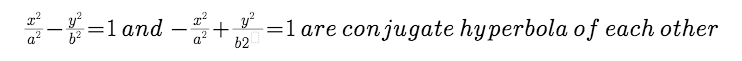FACT :

(i).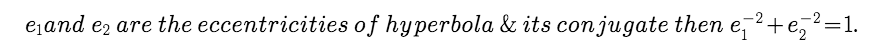(ii). The foci of a hyperbola and its conjugate are concyclic and form the vertices of a square.

(iii) .Two hyperbolas are said to be similar if they have the same eccentricity.

Example :

Question 1:

The eccentricity of the conjugate hyperbola to the hyperbola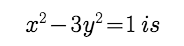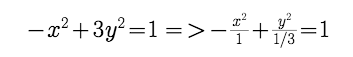Here :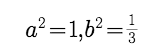eccentricity e =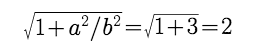RECTANGULAR OR EQUILATERAL HYPERBOLA :

The particular kind of hyperbola in which the lengths of the transverse & conjugate axis are equal is called an

Equilateral Hyperbola. Note that the eccentricity of the rectangular hyperbola is 2 and the length of it's latus rectum

is equal to it's transverse or conjugate axis.

Post By : Devendra Yadav 12 Apr, 2019 1804 views Maths